Tamilnadu State Board New Syllabus Samacheer Kalvi 12th Maths Guide Pdf Chapter 1 Applications of Matrices and Determinants Ex 1.1 Textbook Questions and Answers, Notes.

## Tamilnadu Samacheer Kalvi 12th Maths Solutions Chapter 1 Applications of Matrices and Determinants Ex 1.1

Question 1.
Find the adjoint of the following: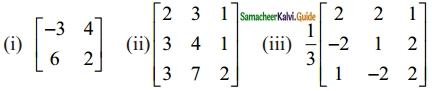Solution: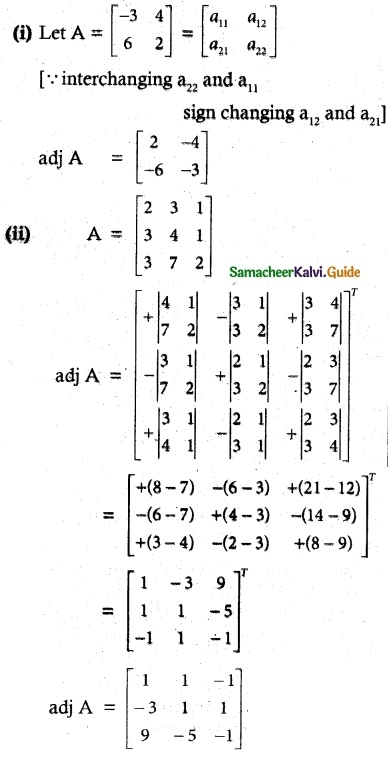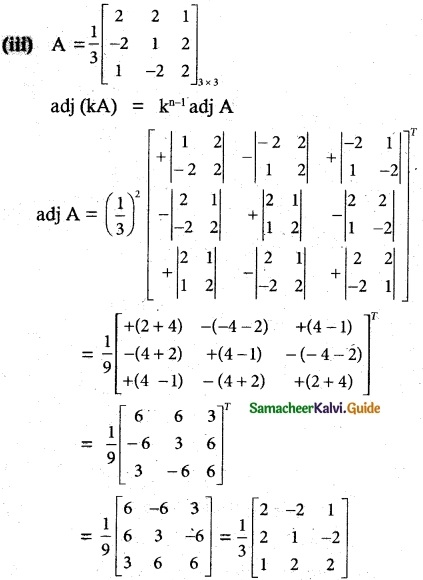Question 2.
Find the inverse (if it exists) of the following.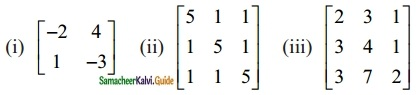Solution:
$$\begin{bmatrix} -2 & 4 \\ 1 & -3 \end{bmatrix}$$
|A| = 6 – 4 = 2 ≠ 0
∴ A-1 exists. A is non singular.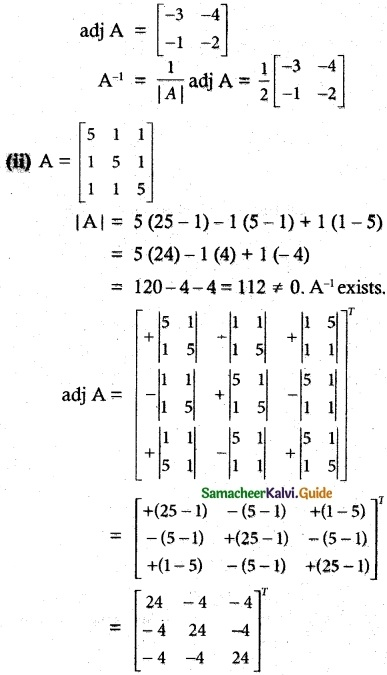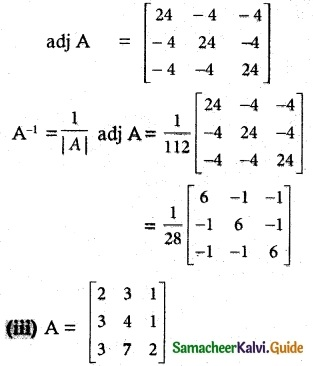|A| = 2(8-7)-3(6-3)+1(21-12)
= 2 – 9 + 9 = 2 ≠ 0. A-1 exists.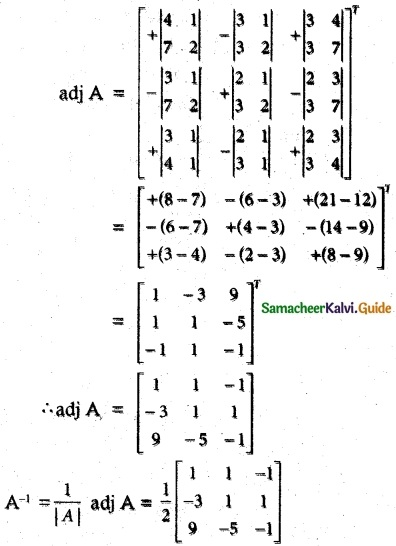Question 3.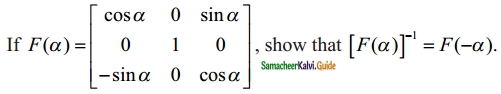Solution:
$$\left[\begin{array}{ccc} \cos \alpha & 0 & \sin \alpha \\ 0 & 1 & 0 \\ -\sin \alpha & 0 & \cos \alpha \end{array}\right]$$
|F(α)| = cos α(cos α – 0) – 0 + sin α(0 + sin α)
= cos²α + sin²α = 1
|f(α)| = 1 ≠ 0. [F(α)]-1 exists.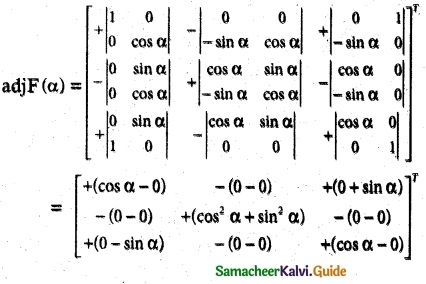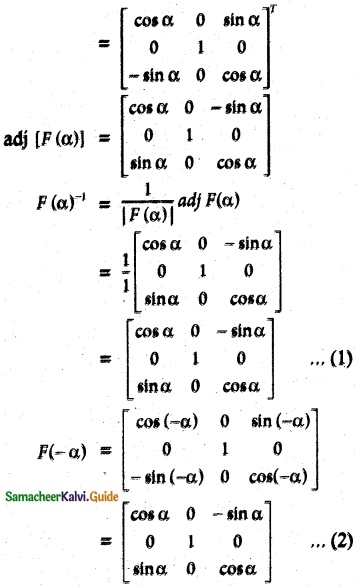[∵ cos (-θ) = cos θ ; sin(-θ) = -sin θ]
from (1) and (2) we have
[F(α)]-1 = F(-α)Question 4.
If A = $$\begin{bmatrix} 5 & 3 \\ -1 & -2 \end{bmatrix}$$, show that A² – 3A – 7I2 = O2. hence find A-1
Solution: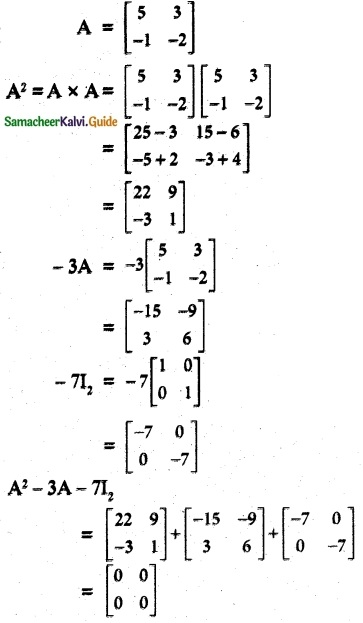∴ A² -3A – 7I2 = O2
Post multiply this equation by A-1
A2A-1 – 3A A-1 – 7I2 A-1 = 0
A – 3I – 7A-1 = 0
A – 3I = 7 A-1
A-1 = $$\frac {1}{7}$$ (A – 3I)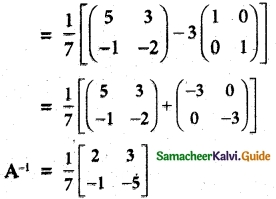Question 5.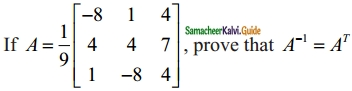Solution: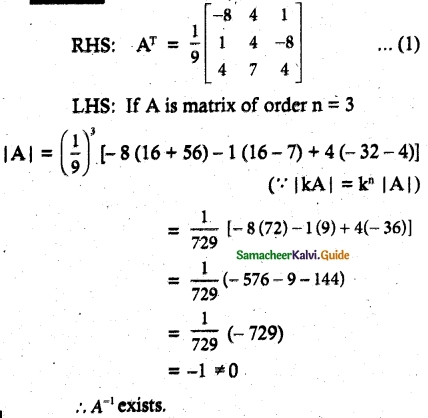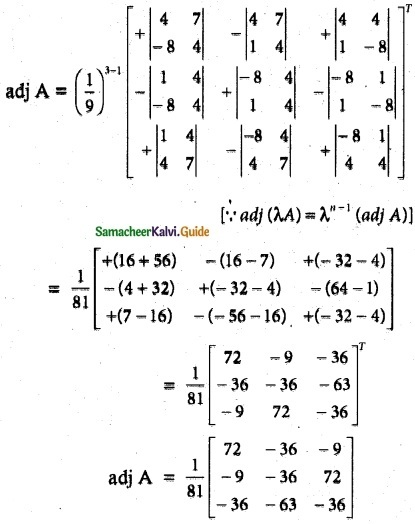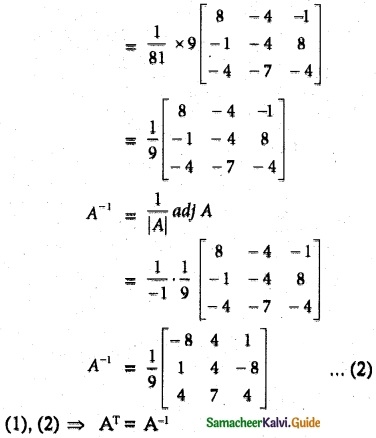Question 6.
If A = $$\begin{bmatrix} 8 & -4 \\ -5 & 3 \end{bmatrix}$$ verify that A(adj A) = (adj A) A = $$\left| A \right|$$I2.
Solution: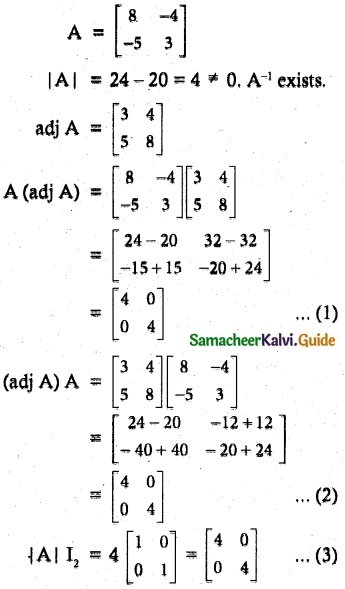(1), (2) and (3) ⇒ A (adj A) = (adj A)A = |A| I2.

Question 7.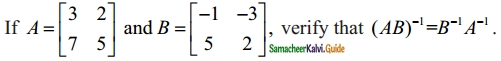Solution: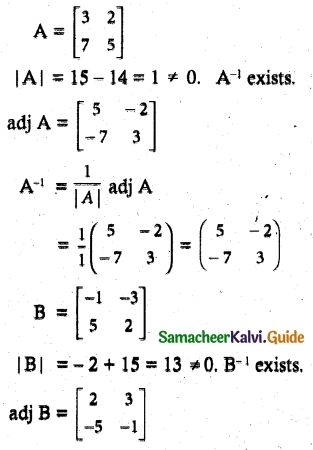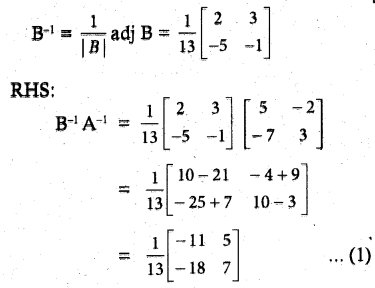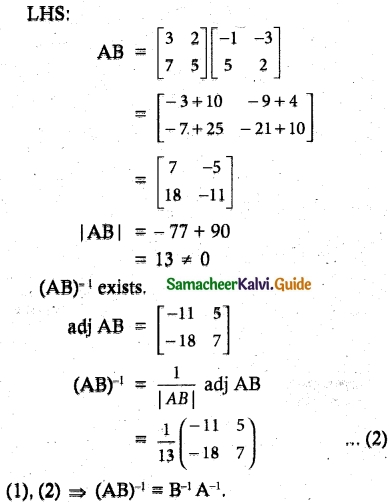Question 8.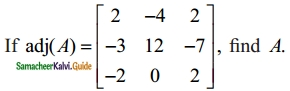Solution: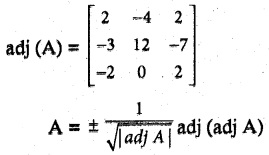|adj (A)| = 2 (24 – 0) + 4 (- 6 – 14) + 2(0 + 24)
= 48 – 80 + 48 = 16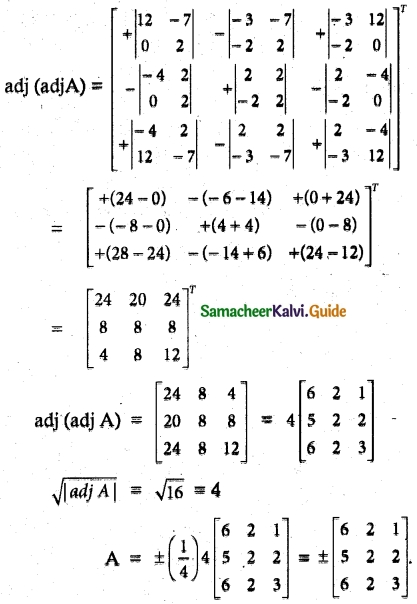Question 9.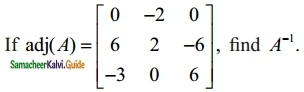Solution: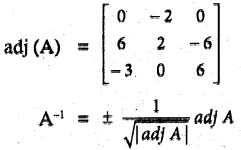|adj A| = 0 + 2(36 – 18) + 0 = 2(18) = 36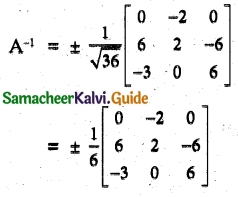Question 10.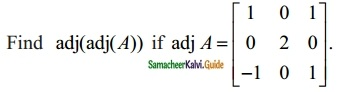Solution: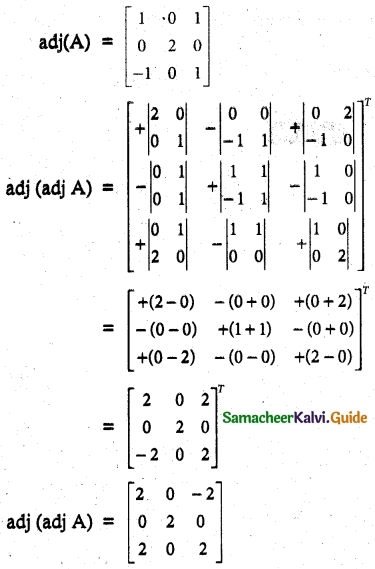Question 11.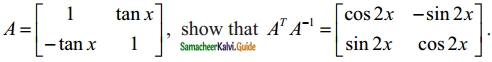Solution: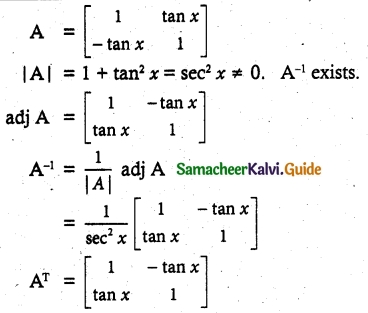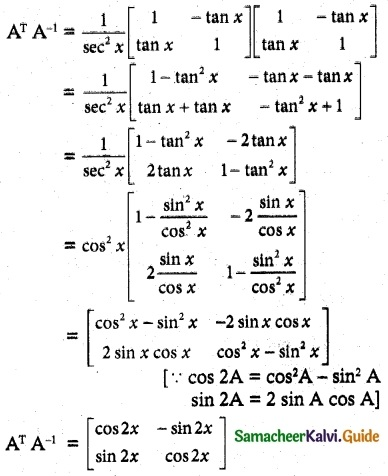Hence provedQuestion 12.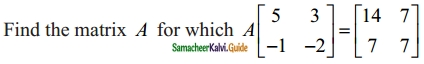Solution: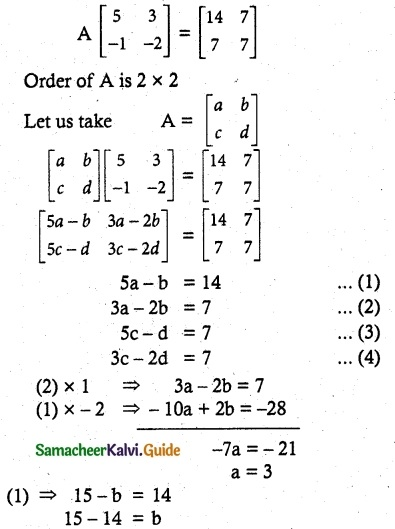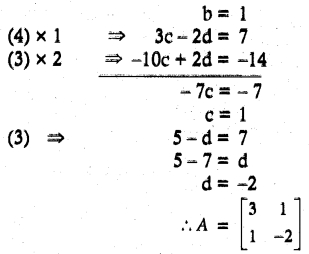Question 13.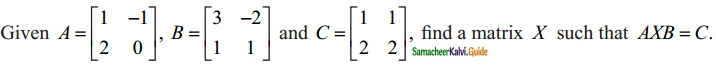Solution:
Given A × B × C
⇒ A-1 A × B B-1 = A-1 C B-1
I × I = A-1 C B-1
⇒ X = A-1 CB-1
let us find A-1 and B-1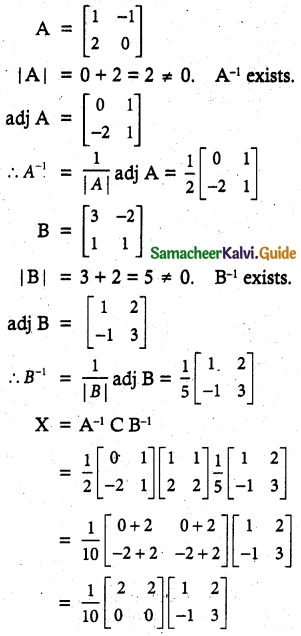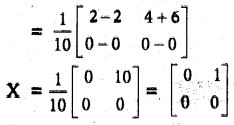Question 14.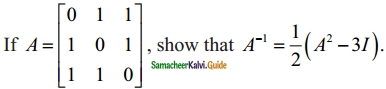Solution: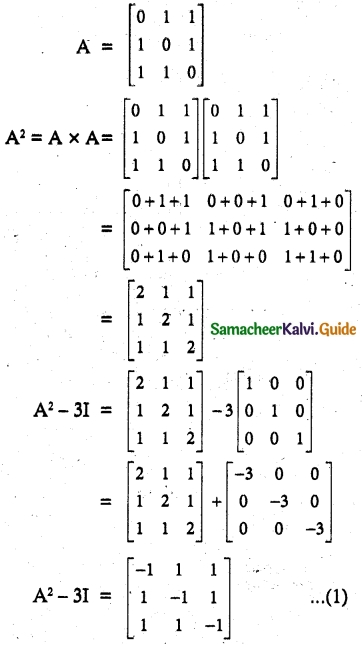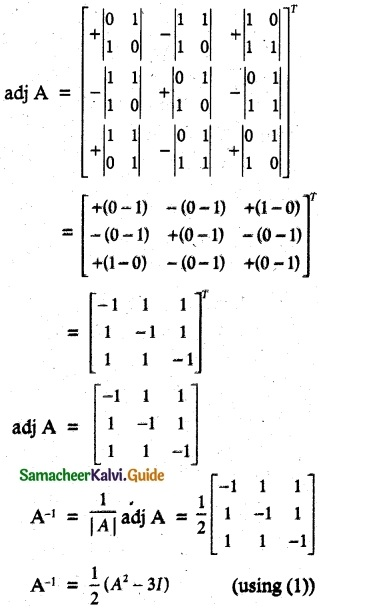Hence proved.Question 15.
Decrypt the received encoded message [2 -3] [20 4] with the encryption matrix $$\begin{bmatrix} -1 & -1 \\ 2 & 1 \end{bmatrix}$$ and the decryption matrix as its inverse, where the system of codes are described by the numbers 1 – 26 to the letters A – Z respectively, and the number 0 to a blank space.
Solution: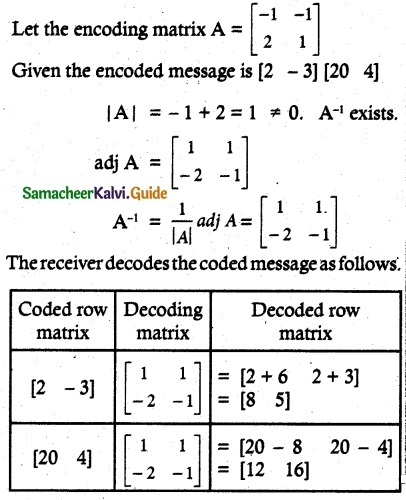So the sequence of decoded row matrics is [8 5] [12 16]